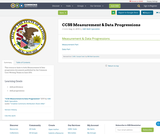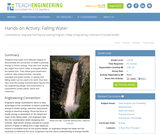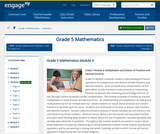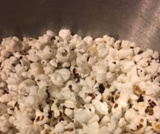# 4 Results

View
Selected filters:
• MCCRS.Math.Content.5.MD.B.2
RemixConditions of Use:
Remix and Share
Rating

This resource links to both Measurement & Data progression documents published by the Common Core Writing Teams in June 2011.

Material Type:
Assessment
Homework/Assignment
Teaching/Learning Strategy
Author:
ISBE Math Specialists
08/08/2019Conditions of Use:
Rating

Students drop water from different heights to demonstrate the conversion of water's potential energy to kinetic energy. They see how varying the height from which water is dropped affects the splash size. They follow good experiment protocol, take measurements, calculate averages and graph results. In seeing how falling water can be used to do work, they also learn how this energy transformation figures into the engineering design and construction of hydroelectric power plants, dams and reservoirs.

Subject:
Engineering
Material Type:
Activity/Lab
Lesson Plan
Provider:
TeachEngineering
Provider Set:
TeachEngineering
Author:
Denise Carlson
Malinda Schaefer Zarske
Natalie Mach
Sabre Duren
Xochitl Zamora-Thompson
09/26/2008Conditions of Use:
Remix and Share
Rating

Grade 5s Module 4 extends student understanding of fraction operations to multiplication and division of both fractions and decimal fractions.  Work proceeds from interpretation of line plots which include fractional measurements to interpreting fractions as division and reasoning about finding fractions of sets through fraction by whole number multiplication.  The module proceeds to fraction by fraction multiplication in both fraction and decimal forms.  An understanding of multiplication as scaling and multiplication by n/n as multiplication by 1 allows students to reason about products and convert fractions to decimals and vice versa.  Students are introduced to the work of division with fractions and decimal fractions.  Division cases are limited to division of whole numbers by unit fractions and unit fractions by whole numbers.  Decimal fraction divisors are introduced and equivalent fraction and place value thinking allow student to reason about the size of quotients, calculate quotients and sensibly place decimals in quotients.  Throughout the module students are asked to reason about these important concepts by interpreting numerical expressions which include fraction and decimal operations and by persevering in solving real-world, multistep problems which include all fraction operations supported by the use of tape diagrams.

Subject:
Ratios and Proportions
Material Type:
Module
Provider:
New York State Education Department
Provider Set:
EngageNY
11/15/2013Conditions of Use:
Remix and Share
Rating

Popcorn pops due to the moisture in the kernel that explodes when heated.  In this lesson students will compare kernels soaked in water before popping with dry kernels to see whether it makes a difference in the number of unpopped kernels.

Subject:
Measurement and Data
Physical Science
Material Type:
Lesson Plan
Author:
Melissa Thiel-Stinson